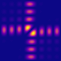QPMS Electromagnetic multiple scattering library and toolkit.
Using QPMS library for simulating finite systems

*** This tutorial is partly obsolete, the interpolators are no longer the first choice of getting the T-matrices. ***

The main C API for finite systems is defined in scatsystem.h, and the most relevant parts are wrapped into python modules. The central data structure defining the system of scatterers is qpms_scatsys_t, which holds information about particle positions and their T-matrices (provided by user) and about the symmetries of the system. Specifically, it keeps track about the symmetry group and how the particles transform under the symmetry operations.

# SVD of a finite symmetric system of scatterers

Let's have look how thinks are done on a small python script. The following script is located in misc/201903_finiterectlat_AaroBEC.py.

#!/usr/bin/env python
from qpms import Particle, CTMatrix, BaseSpec, FinitePointGroup, ScatteringSystem, TMatrixInterpolator, eV, hbar, c
from qpms.symmetries import point_group_info
import numpy as np
import os
import sys
nm = 1e-9
sym = FinitePointGroup(point_group_info['D2h'])
bspec = BaseSpec(lMax = 2)
tmfile = '/m/phys/project/qd/Marek/tmatrix-experiments/Cylinder/AaroBEC/cylinder_50nm_lMax4_cleaned.TMatrix'
interp = TMatrixInterpolator(tmfile, bspec, symmetrise = sym, atol = 1e-8)
# There is only one t-matrix in the system for each frequency. We initialize the matrix with the lowest frequency data.
# Later, we can replace it using the tmatrix[...] = interp(freq) and s.update_tmatrices NOT YET; TODO
omega = float(sys.argv) * eV/hbar
sv_threshold = float(sys.argv)
# Now place the particles and set background index.
px = 571*nm; py = 621*nm
n = 1.51
Nx = int(sys.argv)
Ny = int(sys.argv)
orig_x = (np.arange(Nx/2) + (0 if (Nx % 2) else .5)) * px
orig_y = (np.arange(Ny/2) + (0 if (Ny % 2) else .5)) * py
orig_xy = np.stack(np.meshgrid(orig_x, orig_y), axis = -1)
tmatrix = interp(omega)
particles = [Particle(orig_xy[i], tmatrix) for i in np.ndindex(orig_xy.shape[:-1])]
ss = ScatteringSystem(particles, sym)
k = n * omega / c
for iri in range(ss.nirreps):
mm_iri = ss.modeproblem_matrix_packed(k, iri)
U, S, Vh = np.linalg.svd(mm_iri)
print(iri, ss.irrep_names[iri], S[-1])
starti = max(0,len(S) - np.searchsorted(S[::-1], sv_threshold, side='left')-1)
S=S[starti:], omega=omega, Vh = Vh[starti:], iri=iri, Nx = Nx, Ny= Ny )
# Don't forget to conjugate Vh before transforming it to the full vector!
Definition: symmetries.py:1

Let's have a look at the imports.

from qpms import Particle, CTMatrix, BaseSpec, FinitePointGroup, ScatteringSystem, TMatrixInterpolator, eV, hbar, c
from qpms.symmetries import point_group_info
• Particle is a wrapper over the C structure qpms_particle_t, containing information about particle position and T-matrix.
• CTMatrix is a wrapper over the C structure qpms_tmatrix_t, containing a T-matrix.
• BaseSpec is a wrapper over the C structure qpms_vswf_set_spec_t, defining with which subset of VSWFs we are working with and how their respective coefficients are ordered in memory. Typically, this just means having all electric and magnetic VSWFs up to a given multipole order lMax in the "standard" ordering, but other ways are possible. Note that different Particles (or, more specifically, CTMatrixes) can have different BaseSpecs and happily coexist in the same ScatteringSystem. This makes sense if the system contains particles with different sizes, where the larger particles need cutoff at higher multipole orders.
• FinitePointGroup is a wrapper over the C structure qpms_finite_group_t containing info about a 3D point group and its representation. Its contents are currently not generated using C code. Rather, it is populated using a SVWFPointGroupInfo instance from the point_group_info python dictionary, which uses sympy to generate the group and its representation from generators and some metadata.
• ScatteringSystem is a wrapper over the C structure qpms_scatsys_t, mentioned earlier, containing info about the whole structure.
• TMatrixInterpolator in a wrapper over the C structure qpms_tmatrix_interpolator_t which contains tabulated T-matrices (calculated e.g. using scuff-tmatrix) and generates frequency-interpolated T-matrices based on these.
• eV, hbar, c are numerical constants with rather obvious meanings.

Let's go on:

sym = FinitePointGroup(point_group_info['D2h'])
bspec = BaseSpec(lMax = 2)
tmfile = '/m/phys/project/qd/Marek/tmatrix-experiments/Cylinder/AaroBEC/cylinder_50nm_lMax4_cleaned.TMatrix'
...
interp = TMatrixInterpolator(tmfile, bspec, symmetrise = sym, atol = 1e-8)

The D2h choice indicates that our system will have mirror symmetries along the xy, xz and yz axes. Using the BaseSpec with the standard constructor with lMax = 2 we declare that we include all the VSWFs up to quadrupole order. Next, we create a TMatrixInterpolator based on a file created by scuff-tmatrix. We force the symmetrisation of the T-matrices with the same point group as the overall system symmetry in order to eliminate the possible asymmetries caused by the used mesh. The atol parameter just says that if the absolute value of a given T-matrix element is smaller than the atol value, it is set to zero.

omega = float(sys.argv) * eV/hbar
sv_threshold = float(sys.argv)
# Now place the particles and set background index.
px = 571*nm; py = 621*nm
n = 1.51
Nx = int(sys.argv)
Ny = int(sys.argv)
orig_x = (np.arange(Nx/2) + (0 if (Nx % 2) else .5)) * px
orig_y = (np.arange(Ny/2) + (0 if (Ny % 2) else .5)) * py
orig_xy = np.stack(np.meshgrid(orig_x, orig_y), axis = -1)
tmatrix = interp(omega)
particles = [Particle(orig_xy[i], tmatrix) for i in np.ndindex(orig_xy.shape[:-1])]
ss = ScatteringSystem(particles, sym)

This chunk sets the light frequency and array size based on a command line argument. Then it generates a list of particles covering a quarter of a rectangular array. Finally, these particles are used to generate the final scattering system – the rest of the particles is generated automatically to satisfy the specified system symmetry.

for iri in range(ss.nirreps):
mm_iri = ss.modeproblem_matrix_packed(k, iri)
U, S, Vh = np.linalg.svd(mm_iri)
print(iri, ss.irrep_names[iri], S[-1])
starti = max(0,len(S) - np.searchsorted(S[::-1], sv_threshold, side='left')-1)
The last part iterates over the irreducible representations of the systems. It generates scattering problem LHS (TODO ref) matrix reduced (projected) onto each irrep, and performs SVD on that reduced matrix, and saving the lowest singular values (or all singular values smaller than sv_threshold) together with their respective singular vectors to files.
Examples of how the data generated above can be analysed can be seen in the jupyter notebooks from the qpms_ipynotebooks repository in the AaroBEC directory.